# A posteriori distribution

(diff) ← Older revision | Latest revision (diff) | Newer revision → (diff)

A conditional probability distribution of a random variable, to be contrasted with its unconditional or a priori distribution.

Letbe a random parameter with an a priori density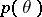, letbe a random result of observations and letbe the conditional density ofwhen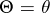; then the a posteriori distribution offor a given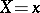, according to the Bayes formula, has the densityIf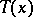is a sufficient statistic for the family of distributions with densities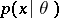, then the a posteriori distribution depends not onitself, but on. The asymptotic behaviour of the a posteriori distributionas, whereare the results of independent observations with density, is "almost independent" of the a priori distribution of.

For the role played by a posteriori distributions in the theory of statistical decisions, see Bayesian approach.

How to Cite This Entry:
A posteriori distribution. Encyclopedia of Mathematics. URL: http://encyclopediaofmath.org/index.php?title=A_posteriori_distribution&oldid=11777
This article was adapted from an original article by Yu.V. Prokhorov (originator), which appeared in Encyclopedia of Mathematics - ISBN 1402006098. See original article